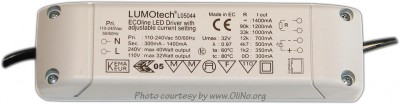# OliNo

Renewable Energy

## LEDcentre – Led Spot 43D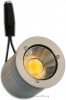presents a led spot lamp. It has a big ledchip emitting warm white light. The current driver used gives a constant current of 1000 mA. Normally there are two ledlamps corrected to this driver, and that is the way this ledlamp is tested (with two lamps on one current driver).

This article shows the measurement results. Many parameters are also found in the Eulumdat file.

See this overview for a comparison with other light bulbs.

### Summary measurement data

parameter meas. result remark
Color temperature 2762 K Warm white
Luminous intensity Iv 542 Cd Measured straight underneath the lamp.
Beam angle 43 deg 43º for all C-planes since the lamp is symmetrical along its 1st axis.
Power P 13.2 W Two led lamps were connected to the current driver which is the most occurring situation. The total power drawn was 26.3 W and this is divided by two to report the power for each lamp.
Power Factor 0.97 For every 1 kWh net power consumed, there has been 0.3 kVAhr for reactive power.
Luminous flux 425 Lm
Luminous efficacy 32 Lm/W
CRI_Ra 93 Color Rendering Index.
Coordinates chromaticity diagram x=0.4570 and y=0.4130
Fitting 230V This lamp is connected to the 230 V grid voltage via a current driver.
PAR-value 5.9 μMol/s/m2 The number of photons seen by an average plant when it is lit by the light of this light bulb. Value valid at 1 m distance from light bulb.
S/P ratio 1.3 This factor indicates the amount of times more efficient the light of this light bulb is perceived under scotopic circumstances (low environmental light level).
L x D external dimensions 50 x 56 mm External dimensions of the lamp.
D luminous area 32 mm Dimensions of the luminous area (used in Eulumdat file). This is the surface of the end of the reflector, at the front of the lamp.
General remarks The ambient temperature during the whole set of measurements was 23.5-24.5 deg C. The temperature of the housing directly around the leds get about 65 degrees hotter than ambient temperature.
Warm up effect: during the warm up time the illuminance decreased with 12 %.

Voltage dependency: the power consumption and illuminance vary insignificantly when the power voltage varies between 200-250 V.

At the end of the article an additional photo is given of the current driver.

Measurement report (PDF)Eulumdat fileRight click on icon and save the file.

### Overview table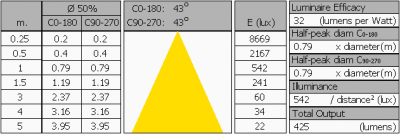The overview table is explained on the OliNo website. Please note that this overview table makes use of calculations, use this data with care as explained on the OliNo site. E (lux) values are not accurate, when within 5 x 32 mm ≈ 160 mm. Within this distance from the lamp, the measured lux values willl be less than the computed values in this overview as the measurements are then within the near field of the lamp.

### Eulumdat light diagram

This light diagram below comes from the program Qlumedit, that extracts these diagrams from an Eulumdat file. It is explained on the OliNo site.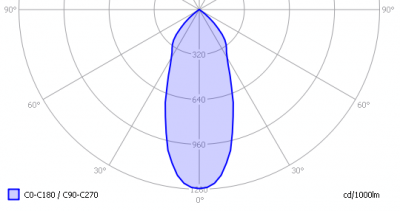The light diagram giving the radiation pattern.

It indicates the luminous intensity around the light bulb. All the planes give the same results as the lamp is symmetrical along its 1st axis.

### Illuminance Ev at 1 m distance, or luminous intensity Iv

Herewith the plot of the averaged luminous intensity Iv as a function of the inclination angle with the light bulb.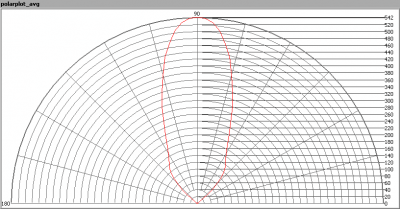The radiation pattern of the light bulb.

This radiation pattern is the average of the light output of the light diagram given earlier. Also, in this graph the luminous intensity is given in Cd.

These averaged values are used (later) to compute the lumen output.Intensity data of every measured turn angle at each inclination angle.

This plot shows per inclination angle the intensity measurement results for each turn angle at that inclination angle. There normally are differences in illuminance values for different turn angles. However for further calculations the averaged values will be used.

When using the average values per inclination angle, the beam angle can be computed, being 43º for all C-planes looked at.

### Luminous flux

With the averaged illuminance data at 1 m distance, taken from the graph showing the averaged radiation pattern, it is possible to compute the luminous flux.

The result of this computation for this light spot is a luminous flux of 425 Lm.

### Luminous efficacy

The luminous flux being 425 Lm, and the power of the light bulb being 13.2 W, yields a luminous efficacy of 32 Lm/W.

### Electrical properties

A power factor of 0.97 means that for every 1 kWh net power consumed, a reactive component of 0.3 kVAr was needed.

 Lamp voltage 230 VAC Lamp current 59 mA Power P 13.2 W Apparent power S 13.6 VA Power factor 0.97

Of this light bulb the voltage across ad the resulting current through it are measured and graphed. See the OliNo site how this is obtained.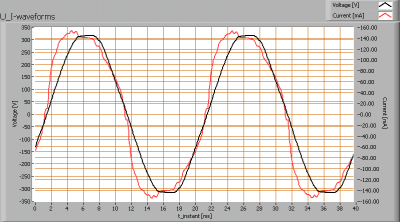Voltage across and current through the lightbulb

The current has a form of a nice sine without a lot of peaks in it. The PF is perfect with a value of 0.97.

When the powerspectrum is made of the current, only a few harmonics are present.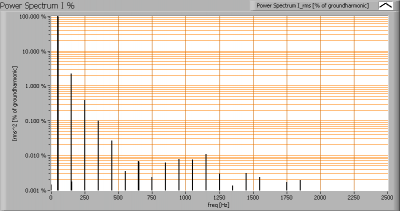The squared terms of the current power spectrum, on a logarithmic scale (in % of the 1st harmonic)

Due to some small irregularities in the sine form of the current a few harmonics are present.

The Total Harmonic Distortion of the current is computed as 17 %.

### Temperature measurements lamp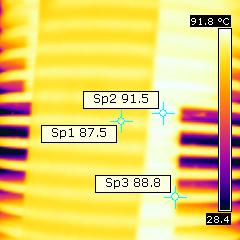Temperature image of the lamp after a long warmup time

 status lamp > 2 hours on ambient temperature 24 deg C reflected background temperature 24 deg C camera Flir BCAM Western S emissivity 0.95(1) measurement distance 0.1 m IFOVgeometric 0.4 mm NETD (thermal sensitivity) 100 mK

(1) The emissivity is set equal to the masking tape that is being used.

SP3 is a measurement directly on the heatsink material. Its value is very close to the value measured on the masking tape hence the emissivities of the heatsink material and that of the masking tape are almost equal.

SP2 is a measurement between the fins of the heatsink. This can be approximated as a black radiator (when the depth between the fins is deeper than 5 times the width between the fins) with an emissivity of > 0.95. When 0.98 is used for instance, the SP2 temperature will be 90 deg C.

The lamp gets hot, but a lot less hot than a regular halogen lamp.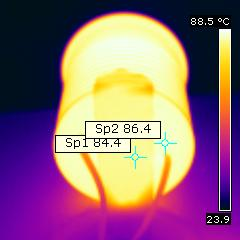High temperatures on the housing.

Emissivity of heatsink material comparable to that of masking tape. This is good, as the heatsink material can easily get rid of thermal energy by radiation.

### Color temperature and Spectral power distributionThe spectral power distribution of this light bulb, energies on y-axis valid at 1 m distance.

The measured color temperature is about 2750 K which is warm white.

This color temperature is measured straight underneath the light bulb. Below a graph showing the color temperature for different inclination angles.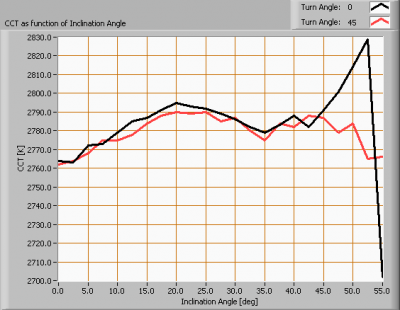Color temperature as a function of inclination angle.

The measurement of CCT is measured for inclination angles up to 55º. Beyond that angle the illuminance was very low (< 5 lux).

The beam angle is 43º, meaning a 21.5º inclination angle. In this area most of the light is present. The variation in correlated color temperature in this area is about 1 %.

### PAR value and PAR spectrum

To make a statement how well the light of this light bulb is for growing plants, the PAR-area needs to be determined. See the OliNo website how this all is determined and the explanation of the graph.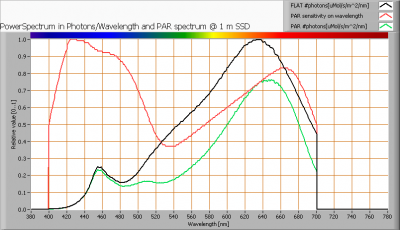The photon spectrum, then the sensitivity curve and as result the final PAR spectrum of the light of this light bulb

parameter value unit
PAR-number 5.9 μMol/s/m²

The PAR efficiency is 65 % (valid for the PAR wave length range of 400 – 700 nm). So maximally 65 % of the total of photons in the light is effectively used by the average plant (since the plant might not take 100 % of the photons at the frequency where its relative sensitivity is 100 %).

### S/P ratio

The S/P ratio and measurement is explained on the OliNo website. Here the results are given.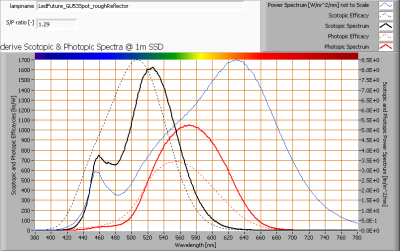The power spectrum, sensitivity curves and resulting scotopic and photopic spectra (spectra energy content defined at 1 m distance).

The S/P ratio is 1.3.

More info on S/P ratio can be found on the OliNo website.

### Chromaticity diagram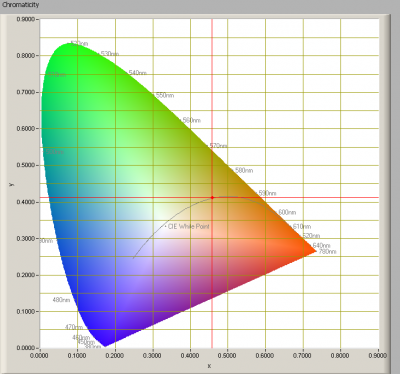The chromaticity space and the position of the lamp’s color coordinates in it.

The light coming from this lamp has its chromaticity coordinates close to the Planckian Locus.

Its coordinates are x=0.4570 and y=0.4130.

### Color Rendering Index (CRI) or also Ra

Herewith the image showing the CRI as well as how well different colors are represented (rendered). The higher the number, the better the resemblance with the color when a black body radiator would have been used (the sun, or an incandescent lamp). Practical information and also some critics about the CRI can be found on the OliNo website.

Each color has an index Rx, and the first 8 indexes (R1 .. R8) are averaged to compute the Ra which is equivalent to the CRI.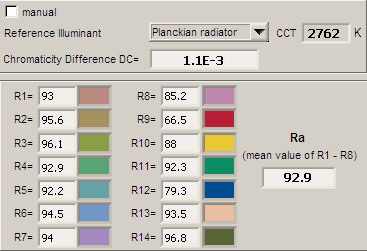CRI of the light of this lightbulb.

The value of 93 is much higher than 80 which is considered a minimum value for indoor usage.

Note: the chromaticity difference is 0.0011 indicates the distance to the Planckian Locus. There is no norm yet that states what the max deviation from white light is allowed to be.

### Voltage dependency

The dependency of a number of lamp parameters on the lamp voltage is determined. For this, the lamp voltage has been varied and its effect on the following light bulb parameters measured: illuminance E_v [lx], the lamppower P [W], the color temperature [K] and the luminous efficacy [Lm/W].Lamp voltage dependencies of certain light bulb parameters, where the value at 230 V is taken as 100 %.

The illuminance and consumed power do not vary significantly when the voltage is varied.

When the voltage at 230 V varies with + and – 5 V, then the illuminance varies < 0.1 %, so when abrupt voltage changes occur this effect is not visible in the illuminance output.

### Warm up effects

After switch on of a cold lamp, the effect of heating up of the lamp is measured on illuminance E_v [lx], the lamppower P [W] and the luminous efficacy [lm/W].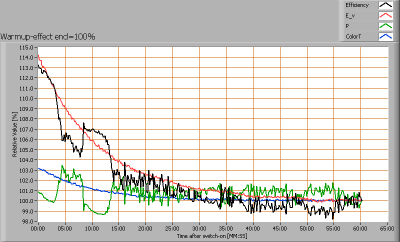Effect of warming up on different light bulb parameters. At top the 100 % level is put at begin, and at bottom at the end.

The warm up time is about 20 minutes. During this time the illuminance decreases with about 12 %.